Test: Network Theorems

# Test: Network Theorems

Test Description

## 10 Questions MCQ Test Analog Electronics | Test: Network Theorems

Test: Network Theorems for Electrical Engineering (EE) 2023 is part of Analog Electronics preparation. The Test: Network Theorems questions and answers have been prepared according to the Electrical Engineering (EE) exam syllabus.The Test: Network Theorems MCQs are made for Electrical Engineering (EE) 2023 Exam. Find important definitions, questions, notes, meanings, examples, exercises, MCQs and online tests for Test: Network Theorems below.
Solutions of Test: Network Theorems questions in English are available as part of our Analog Electronics for Electrical Engineering (EE) & Test: Network Theorems solutions in Hindi for Analog Electronics course. Download more important topics, notes, lectures and mock test series for Electrical Engineering (EE) Exam by signing up for free. Attempt Test: Network Theorems | 10 questions in 10 minutes | Mock test for Electrical Engineering (EE) preparation | Free important questions MCQ to study Analog Electronics for Electrical Engineering (EE) Exam | Download free PDF with solutions
 1 Crore+ students have signed up on EduRev. Have you?
Test: Network Theorems - Question 1

###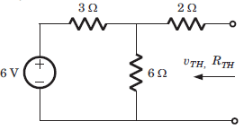Q. Vth = ?

Detailed Solution for Test: Network Theorems - Question 1

Vth = (6)(6)/(6 + 3) or 4 V.

Test: Network Theorems - Question 2

###Q. Rth = ? (in ohm)

Detailed Solution for Test: Network Theorems - Question 2

Rth = (3||6) + 2 or 4 ohm.

Test: Network Theorems - Question 3

### A battery has a short-circuit current of 30 A and an open circuit voltage of 24 V. If the battery is connected to an electric bulb of resistance 2 ohm-1, the power dissipated by the bulb is

Test: Network Theorems - Question 4

Circuit for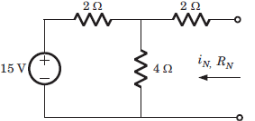Q. In = ?

Detailed Solution for Test: Network Theorems - Question 4

V1 = (15/2)/(1/4 + 1/2 + 1/2)
In = Isc = V1/2 = 3 A.

Test: Network Theorems - Question 5Rn = ? (in ohm)

Detailed Solution for Test: Network Theorems - Question 5

Rn = (2||4) + 2.

Test: Network Theorems - Question 6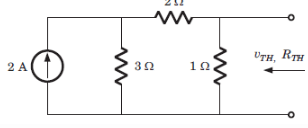Q. Vth = ?

Detailed Solution for Test: Network Theorems - Question 6

Vth = (2)(3)(1)/3+3 = 1V.

Test: Network Theorems - Question 7Q. Rth = ? (in ohm)

Detailed Solution for Test: Network Theorems - Question 7

Rth = 1||5 or 5/6 ohm.

Test: Network Theorems - Question 8

The equivalent to the given circuit is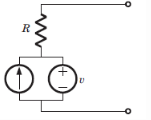Detailed Solution for Test: Network Theorems - Question 8

After killing all source equivalent resistance is R. Open circuit voltage is v1.

Test: Network Theorems - Question 9

V1 = ?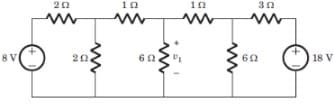Detailed Solution for Test: Network Theorems - Question 9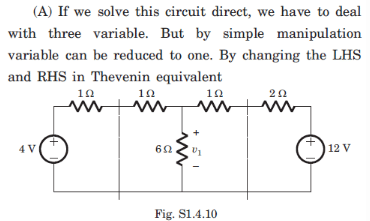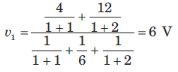Test: Network Theorems - Question 10

i1 = ?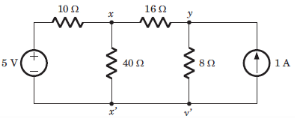Detailed Solution for Test: Network Theorems - Question 10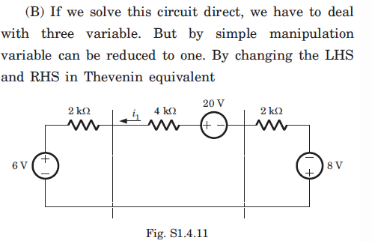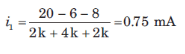## Analog Electronics

11 videos|57 docs|108 tests
Information about Test: Network Theorems Page
In this test you can find the Exam questions for Test: Network Theorems solved & explained in the simplest way possible. Besides giving Questions and answers for Test: Network Theorems, EduRev gives you an ample number of Online tests for practice

## Analog Electronics

11 videos|57 docs|108 tests(Scan QR code)